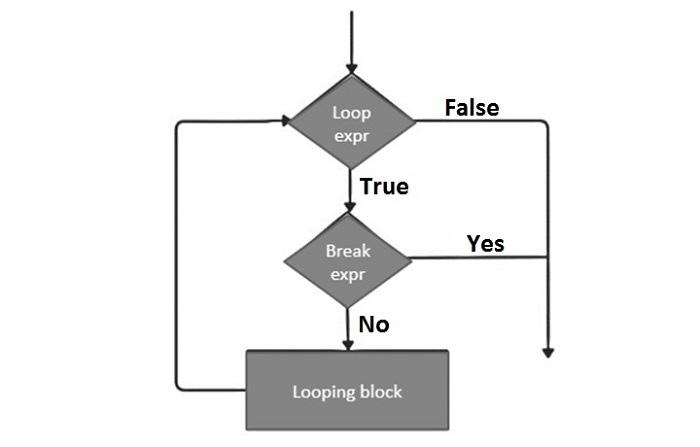# Python - The break Statement

## Loop Control Statements

The Loop control statements change the execution from its normal sequence. When the execution leaves a scope, all automatic objects that were created in that scope are destroyed.

Python supports the following control statements −

Sr.No Control Statement & Description
1

break statement

Terminates the loop statement and transfers execution to the statement immediately following the loop.

2

continue statement

Causes the loop to skip the remainder of its body and immediately retest its condition prior to reiterating.

3

pass statement

The pass statement in Python is used when a statement is required syntactically but you do not want any command or code to execute.

Let us go through the loop control statements briefly.

## Python â€“ The break Statement

The break statement is used for premature termination of the current loop. After abandoning the loop, execution at the next statement is resumed, just like the traditional break statement in C.

The most common use of break is when some external condition is triggered requiring a hasty exit from a loop. The break statement can be used in both while and for loops.

If you are using nested loops, the break statement stops the execution of the innermost loop and starts executing the next line of the code after the block.

### Syntax

The syntax for a break statement in Python is as follows −

```break
```

### Flow Diagram

Its flow diagram looks like this −### Example 1

Now let's take an example to understand how the "break" statement works in Python −

```#!/usr/bin/python3
print ('First example')
for letter in 'Python': # First Example
if letter == 'h':
break
print ('Current Letter :', letter)
print ('Second example')
var = 10 # Second Example
while var > 0:
print ('Current variable value :', var)
var = var -1
if var == 5:
break
print ("Good bye!")
```

When the above code is executed, it produces the following output

```First example
Current Letter : P
Current Letter : y
Current Letter : t
Second example
Current variable value : 10
Current variable value : 9
Current variable value : 8
Current variable value : 7
Current variable value : 6
Good bye!
```

### Example 2

The following program demonstrates the use of break in a for loop iterating over a list. User inputs a number, which is searched in the list. If it is found, then the loop terminates with the 'found' message.

```#!/usr/bin/python3
no=int(input('any number: '))
numbers=[11,33,55,39,55,75,37,21,23,41,13]
for num in numbers:
if num==no:
print ('number found in list')
break
else:
```

The above program will produce the following output

```any number: 33
number found in list
any number: 5
```

### Example 3: Checking for Prime Number

Note that when the break statement is encountered, Python abandons the remaining statements in the loop, including the else block.

The following example takes advantage of this behaviour to find whether a number is prime or not. By definition, a number is prime if it is not divisible by any other number except 1 and itself.

The following code runs a for loop over numbers from 2 to the desired number-1. If it divisible by any value of looping variable, the number is not prime, hence the program breaks from the loop. If the number is not divisible by any number between 2 and x-1, the else block prints the message that the given number is prime.

```num = 37
print ("Number: ", num)
for x in range(2,num):
if num%x==0:
print ("{} is not prime".format(num))
break
else:
print ("{} is prime".format(num))
```

### Output

Assign different values to num to check if it is a prime number or not.

```Number: 37
37 is prime
Number: 49
49 is not prime
```